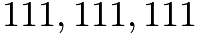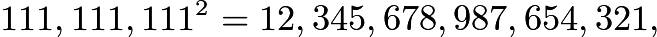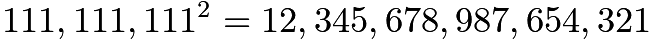# January 2018 Brain Puzzler Solution

Q: What is the sum of the digits of the square of$111,111,111$?

A: 81
Using the standard multiplication algorithm,$111,111,111^2=12,345,678,987,654,321,$whose digit sum is 81.

Or by looking at the pattern:$11^2 = 121 \\ 111^2 = 12321 \\ 1111^2 = 1234321$$111,111,111^2=12,345,678,987,654,321$ whose digit sum is 81
There is a shortcut to adding these digits
Reading from left to right, we can add the first 8 digits, 1 through 8 by making 4 pairs of 9 (1 + 8, 2 + 7, 3 + 6 and 4 + 5) making 36
Reading from right to left, we can add the last 8 digits (1- 8) the same way making 36.
Leaving the middle digit of 9.

36 + 36 + 9 = 81